# 2.4 可变数据

## 2.4.1 局部状态

``````>>> withdraw(25)
75
>>> withdraw(25)
50
>>> withdraw(60)
'Insufficient funds'
>>> withdraw(15)
35
``````

``````>>> withdraw = make_withdraw(100)
``````

`make_withdraw`的实现需要新类型的语句：`nonlocal`语句。当我们调用`make_withdraw`时，我们将名称`balance`绑定到初始值上。之后我们定义并返回了局部函数，`withdraw`，它在调用时更新并返回`balance`的值。

``````>>> def make_withdraw(balance):
"""Return a withdraw function that draws down balance with each call."""
def withdraw(amount):
nonlocal balance                 # Declare the name "balance" nonlocal
if amount > balance:
return 'Insufficient funds'
balance = balance - amount       # Re-bind the existing balance name
return balance
return withdraw
``````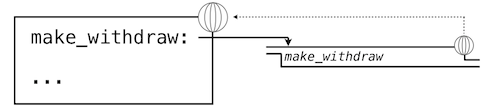``````>>> wd = make_withdraw(20)
``````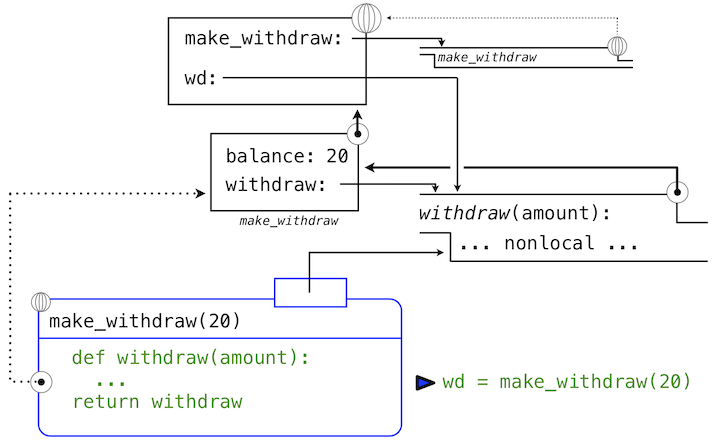``````>>> wd(5)
15
``````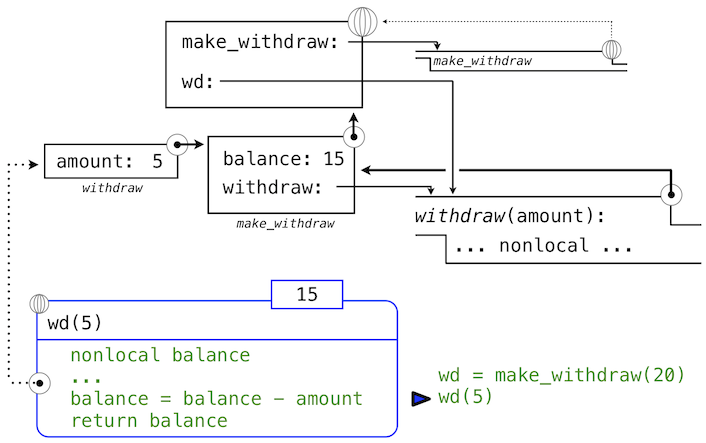`withdraw`的赋值语句通常在`withdraw`的局部帧中为`balance`创建新的绑定。由于`nonlocal`语句，赋值运算找到了`balance`定义位置的第一帧，并在那里重新绑定名称。如果`balance`之前没有绑定到值上，那么`nonlocal`语句会产生错误。

``````>>> wd(3)
12
``````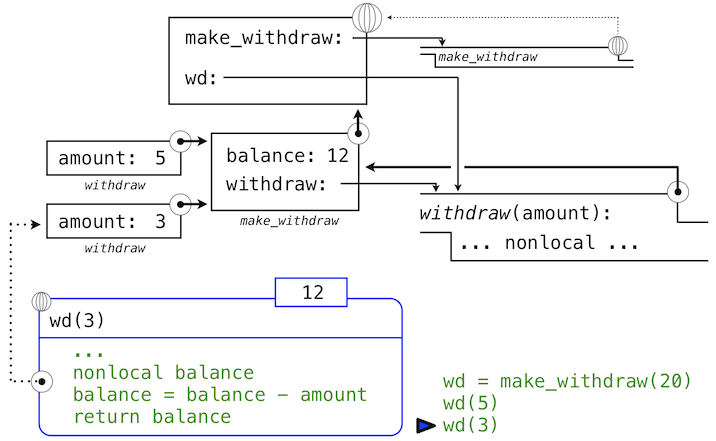## 2.4.2 非局部赋值的好处

``````>>> wd2 = make_withdraw(7)
``````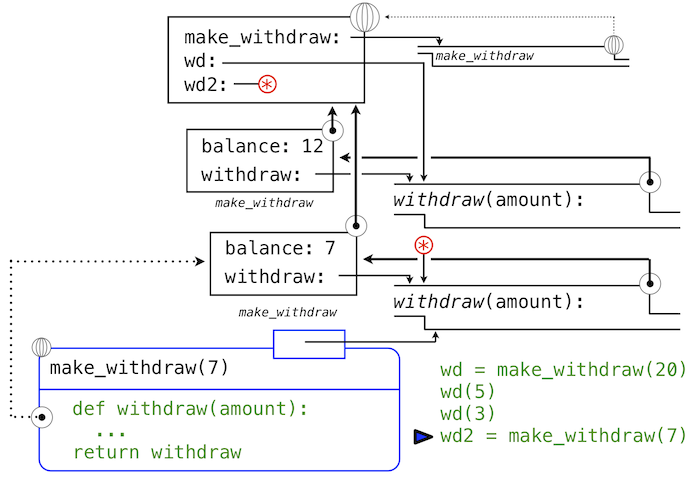``````>>> wd2(6)
1
``````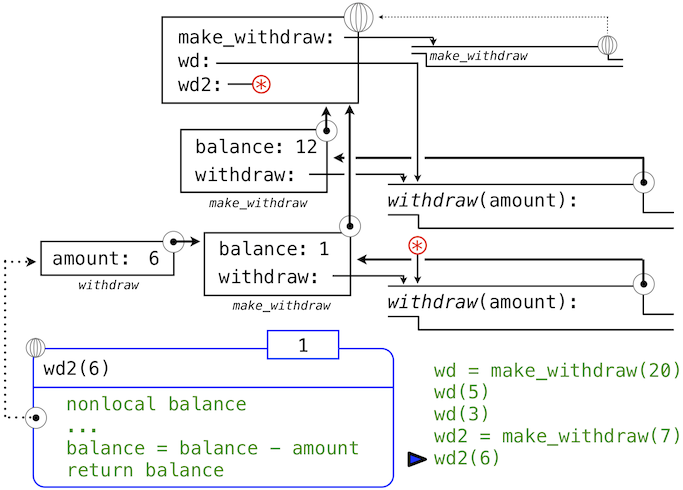## 2.4.3 非局部赋值的代价

``````>>> wd = make_withdraw(12)
>>> wd2 = wd
>>> wd2(1)
11
>>> wd(1)
10
``````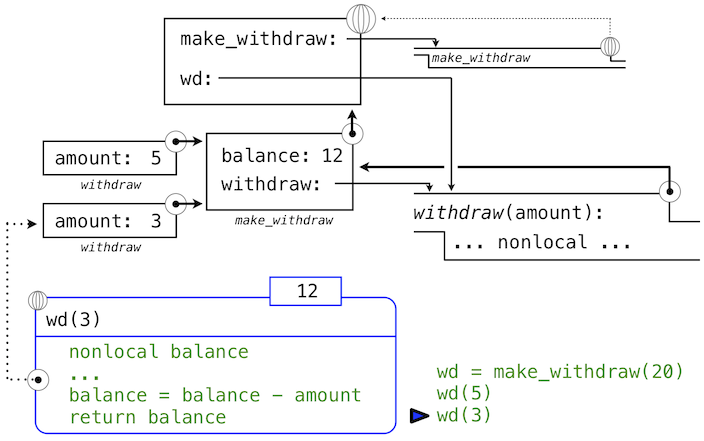## 2.4.4 列表

`list`是 Python 中最使用和灵活的洗了类型。列表类似于元组，但是它是可变的。方法调用和赋值语句都可以修改列表的内容。

``````>>> chinese_suits = ['coin', 'string', 'myriad']  # A list literal
>>> suits = chinese_suits                         # Two names refer to the same list
``````

``````>>> suits.pop()             # Removes and returns the final element
>>> suits.remove('string')  # Removes the first element that equals the argument
``````

``````>>> suits.append('cup')              # Add an element to the end
>>> suits.extend(['sword', 'club'])  # Add all elements of a list to the end
``````

``````>>> suits = 'spade'  # Replace an element
``````

``````>>> suits
``````

``````>>> suits[0:2] = ['heart', 'diamond']  # Replace a slice
>>> suits
``````

``````>>> chinese_suits  # This name co-refers with "suits" to the same list
``````

``````>>> nest = list(suits)  # Bind "nest" to a second list with the same elements
>>> nest = suits     # Create a nested list
``````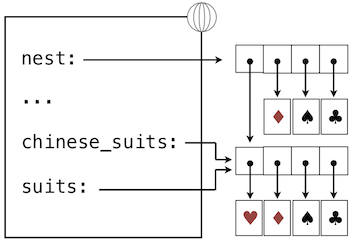``````>>> suits.insert(2, 'Joker')  # Insert an element at index 2, shifting the rest
>>> nest
``````

``````>>> suits is nest
True
>>> suits is ['heart', 'diamond', 'spade', 'club']
False
>>> suits == ['heart', 'diamond', 'spade', 'club']
True
``````

``````>>> from unicodedata import lookup
>>> [lookup('WHITE ' + s.upper() + ' SUIT') for s in suits]
['♡', '♢', '♤', '♧']
``````

``````>>> def make_mutable_rlist():
"""Return a functional implementation of a mutable recursive list."""
contents = empty_rlist
def dispatch(message, value=None):
nonlocal contents
if message == 'len':
return len_rlist(contents)
elif message == 'getitem':
return getitem_rlist(contents, value)
elif message == 'push_first':
contents = make_rlist(value, contents)
elif message == 'pop_first':
f = first(contents)
contents = rest(contents)
return f
elif message == 'str':
return str(contents)
return dispatch
``````

``````>>> def to_mutable_rlist(source):
"""Return a functional list with the same contents as source."""
s = make_mutable_rlist()
for element in reversed(source):
s('push_first', element)
return s
``````

``````>>> s = to_mutable_rlist(suits)
>>> type(s)
<class 'function'>
>>> s('str')
``````

``````>>> s('pop_first')
'heart'
>>> s('str')
``````

## 2.4.5 字典

``````>>> numerals = {'I': 1.0, 'V': 5, 'X': 10}
``````

``````>>> numerals['X']
10
``````

``````>>> numerals['I'] = 1
>>> numerals['L'] = 50
>>> numerals
{'I': 1, 'X': 10, 'L': 50, 'V': 5}
``````

``````>>> sum(numerals.values())
66
``````

``````>>> dict([(3, 9), (4, 16), (5, 25)])
{3: 9, 4: 16, 5: 25}
``````

• 字典的键不能是可变内建类型的对象。
• 一个给定的键最多只能有一个值。

``````>>> numerals.get('A', 0)
0
>>> numerals.get('V', 0)
5
``````

``````>>> {x: x*x for x in range(3,6)}
{3: 9, 4: 16, 5: 25}
``````

``````>>> def make_dict():
"""Return a functional implementation of a dictionary."""
records = []
def getitem(key):
for k, v in records:
if k == key:
return v
def setitem(key, value):
for item in records:
if item == key:
item = value
return
records.append([key, value])
def dispatch(message, key=None, value=None):
if message == 'getitem':
return getitem(key)
elif message == 'setitem':
setitem(key, value)
elif message == 'keys':
return tuple(k for k, _ in records)
elif message == 'values':
return tuple(v for _, v in records)
return dispatch
``````

``````>>> d = make_dict()
>>> d('setitem', 3, 9)
>>> d('setitem', 4, 16)
>>> d('getitem', 3)
9
>>> d('getitem', 4)
16
>>> d('keys')
(3, 4)
>>> d('values')
(9, 16)
``````

## 2.4.6 示例：传播约束

``````p * v = n * k * t
``````

``````9 * c = 5 * (f - 32)
````````````>>> celsius = make_connector('Celsius')
>>> fahrenheit = make_connector('Fahrenheit')
``````

``````>>> def make_converter(c, f):
"""Connect c to f with constraints to convert from Celsius to Fahrenheit."""
u, v, w, x, y = [make_connector() for _ in range(5)]
multiplier(c, w, u)
multiplier(v, x, u)
constant(w, 9)
constant(x, 5)
constant(y, 32)
>>> make_converter(celsius, fahrenheit)
``````

``````>>> celsius['set_val']('user', 25)
Celsius = 25
Fahrenheit = 77.0
``````

``````>>> fahrenheit['set_val']('user', 212)
``````

``````>>> celsius['forget']('user')
Celsius is forgotten
Fahrenheit is forgotten
``````

``````>>> fahrenheit['set_val']('user', 212)
Fahrenheit = 212
Celsius = 100.0
``````

• `connector['set_val'](source, value)` 表示`source`请求连接器将当前值设置为该值。
• `connector['has_val']()` 返回连接器是否已经有了一个值。
• `connector['val']` 是连接器的当前值。
• `connector['forget'](source)` 告诉连接器，`source`请求它忘掉当前值。
• `connector['connect'](source)` 告诉连接器参与新的约束`source`

• `constraint['new_val']()` 表示连接到约束的连接器有了新的值。
• `constraint['forget']()` 表示连接到约束的连接器需要忘掉它的值。

`adder`函数在两个连接器上构造了加法器约束，其中前两个连接器必须加到第三个上：`a + b = c`。为了支持多方向的约束传播，加法器必须也规定从`c`中减去`a`会得到`b`，或者从`c`中减去`b`会得到`a`

``````>>> from operator import add, sub
"""The constraint that a + b = c."""
return make_ternary_constraint(a, b, c, add, sub, sub)
``````

``````>>> def make_ternary_constraint(a, b, c, ab, ca, cb):
"""The constraint that ab(a,b)=c and ca(c,a)=b and cb(c,b) = a."""
def new_value():
av, bv, cv = [connector['has_val']() for connector in (a, b, c)]
if av and bv:
c['set_val'](constraint, ab(a['val'], b['val']))
elif av and cv:
b['set_val'](constraint, ca(c['val'], a['val']))
elif bv and cv:
a['set_val'](constraint, cb(c['val'], b['val']))
def forget_value():
for connector in (a, b, c):
connector['forget'](constraint)
constraint = {'new_val': new_value, 'forget': forget_value}
for connector in (a, b, c):
connector['connect'](constraint)
return constraint
``````

`multiplier``adder`类似：

``````>>> from operator import mul, truediv
>>> def multiplier(a, b, c):
"""The constraint that a * b = c."""
return make_ternary_constraint(a, b, c, mul, truediv, truediv)
``````

``````>>> def constant(connector, value):
"""The constraint that connector = value."""
constraint = {}
connector['set_val'](constraint, value)
return constraint
``````

``````>>> def make_connector(name=None):
"""A connector between constraints."""
informant = None
constraints = []
def set_value(source, value):
nonlocal informant
val = connector['val']
if val is None:
informant, connector['val'] = source, value
if name is not None:
print(name, '=', value)
inform_all_except(source, 'new_val', constraints)
else:
if val != value:
def forget_value(source):
nonlocal informant
if informant == source:
informant, connector['val'] = None, None
if name is not None:
print(name, 'is forgotten')
inform_all_except(source, 'forget', constraints)
connector = {'val': None,
'set_val': set_value,
'forget': forget_value,
'has_val': lambda: connector['val'] is not None,
'connect': lambda source: constraints.append(source)}
return connector
``````

``````>>> def inform_all_except(source, message, constraints):
"""Inform all constraints of the message, except source."""
for c in constraints:
if c != source:
c[message]()
``````

`has_val`消息的响应表示连接器是否拥有一个值。对`connect`消息的响应将`source`约束添加到约束列表中。